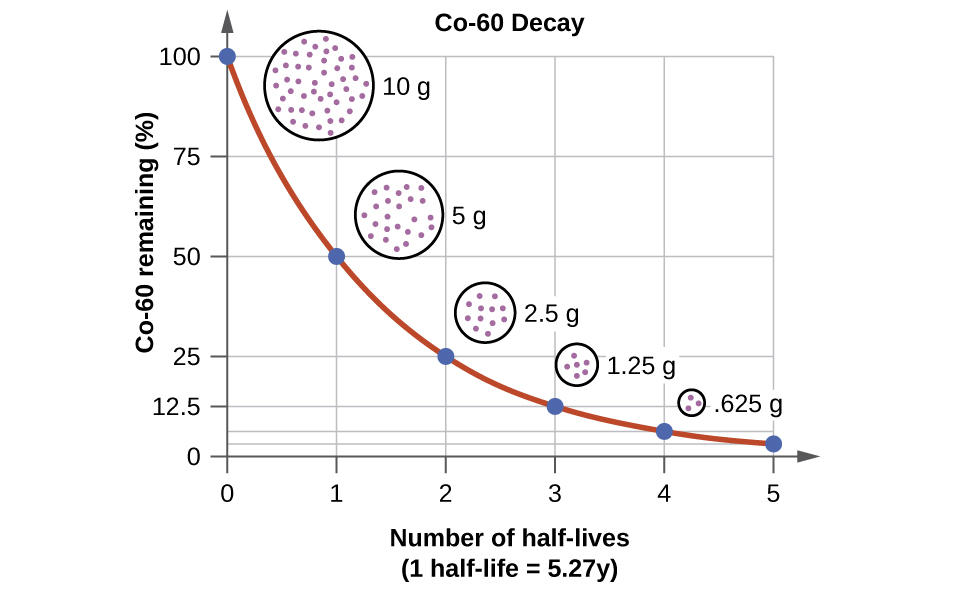Categories

Each radioactive nuclide has a characteristic, constant half-life (t1/2), the time required for half of the atoms in a sample to decay. An isotope’s half-life allows us to determine how long a sample of a useful isotope will be available, and how long a sample of an undesirable or dangerous isotope must be stored before it decays to a low-enough radiation level that is no longer a problem.

For example, cobalt-60, an isotope that emits gamma rays used to treat cancer, has a half-life of 5.27 years (Figure 3.5). In a given cobalt-60 source, since half of the$_{27}^{60}\text{Co}$ nuclei decay every 5.27 years, both the amount of material and the intensity of the radiation emitted is cut in half every 5.27 years. Note that for a given substance, the intensity of radiation that it produces is directly proportional to the rate of decay of the substance and the amount of the substance. Thus, a cobalt-60 source that is used for cancer treatment must be replaced regularly to continue to be effective.The Decay of Cobalt-60. For cobalt-60, which has a half-life of 5.27 years, 50% remains after 5.27 years (one half-life), 25% remains after 10.54 years (two half-lives), 12.5% remains after 15.81 years (three half-lives), and so on. Note that every half-life is the same length of time.

Since every half-life for a radionuclide is the same length of time, we can use the following equation to calculate how much radioactive nuclide is remaining after the passage of any number (n) of half-lives:

## Practice Problem:

Question: The half-life of Zn-71 is 2.4 minutes.  If one had 100.0 g at the beginning, how many grams would be left after 7.2 minutes has elapsed?

Solution:

Step 1. Determine the number of half-lives that have passed:    number of half-lives = time passed divided by the half-life (Be sure that the time units match!!)

Step 2. Use the Isotope Remaining equation to solve for how much isotope will remain after the number of half-lives determined in step 1 have passed.